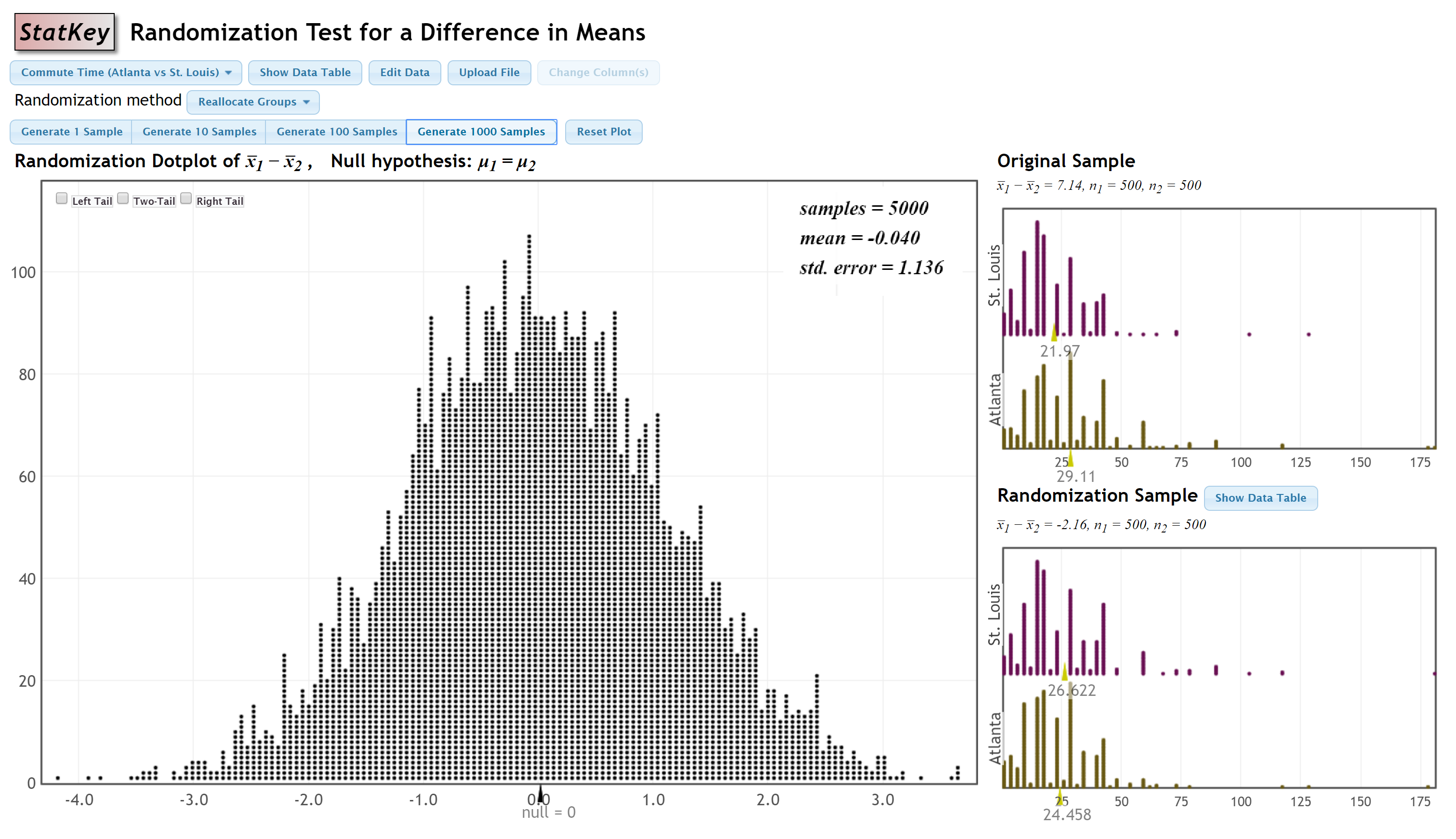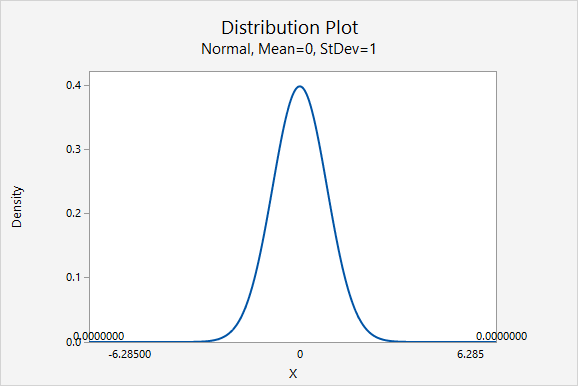# 7.4.1.6 - Example: Difference in Mean Commute Times

Research question: Do the mean commute times in Atlanta and St. Louis differ in the population?

StatKey was used to construct a randomization distribution:#### Step 1: Check assumptions and write hypotheses

From the given StatKey output, the sampling distribution is approximately normal.

$$H_0: \mu_1-\mu_2=0$$

$$H_a: \mu_1 - \mu_2 \ne 0$$

#### Step 2: Compute the test statistic

$$test\;statistic=\dfrac{sample\;statistic - null \; parameter}{standard \;error}$$

The observed sample statistic is $$\overline x _1 - \overline x _2 = 7.14$$. The null parameter is 0. And, the standard error, from the StatKey output, is 1.136.

$$test\;statistic=\dfrac{7.14-0}{1.136}=6.285$$

#### Step 3: Determine the p value

The p value will be the area on the z distribution that is more extreme than the test statistic of 6.285, in the direction of the alternative hypothesis:This was a two-tailed test. The area in the two tailed combined is 0.000000. Theoretically, the p value cannot be 0 because there is always some chance that a Type I error was committed. This p value would be written as p < 0.001.

#### Step 4: Make a decision

The p value is smaller than the standard 0.05 alpha level, therefore we reject the null hypothesis.

#### Step 5: State a "real world" conclusion

There is evidence that the mean commute times in Atlanta and St. Louis are different in the population.